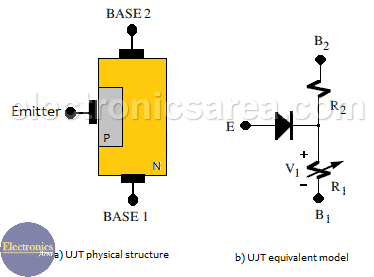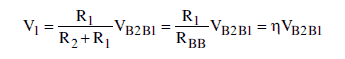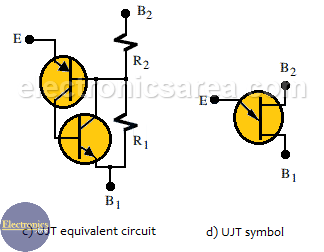# Unijunction transistor – UJT

The UJT or Unijunction transistor is constituted by two polluted regions, with three external terminals: two bases and one emitter. In the (a) image, the physical structure of this device is shown. The emitter is strongly doped with “p” impurities and the n region weakly doped with “n” impurities.

Therefore, the resistance between the two bases, RBB or interbase resistance, is high (from 5K to 10K when the emitter is open).

## UJT Physical Structure and Equivalent Model

The equivalent model represented in the (b) image is constituted by a diode that excites the two internal resistor’s junction, R1 and R2, which verify that RBB = R1 + R2.When the diode does not conduct, the voltage drop on the R1 resistor (V1) can be expressed as:where:

• VB2B1 is the voltage difference between the bases of the UJT and …
• The voltage division factor known as the intrinsic relation = R1/(R2+R1) = R1/RBB

## UJT – Unijunction transistor equivalent circuit and symbol

The model of this device using transistors is shown in image (c). Its structure is very similar to the four-layer diode structure. When the transistors go into conduction, the voltage drop in R is very low. The symbol of the UJT is shown in the image (d).• 3
•
•
•
•
•
•
•
•
3
Shares
•
3
Shares
• 3
•
•
•
•
•
•
•